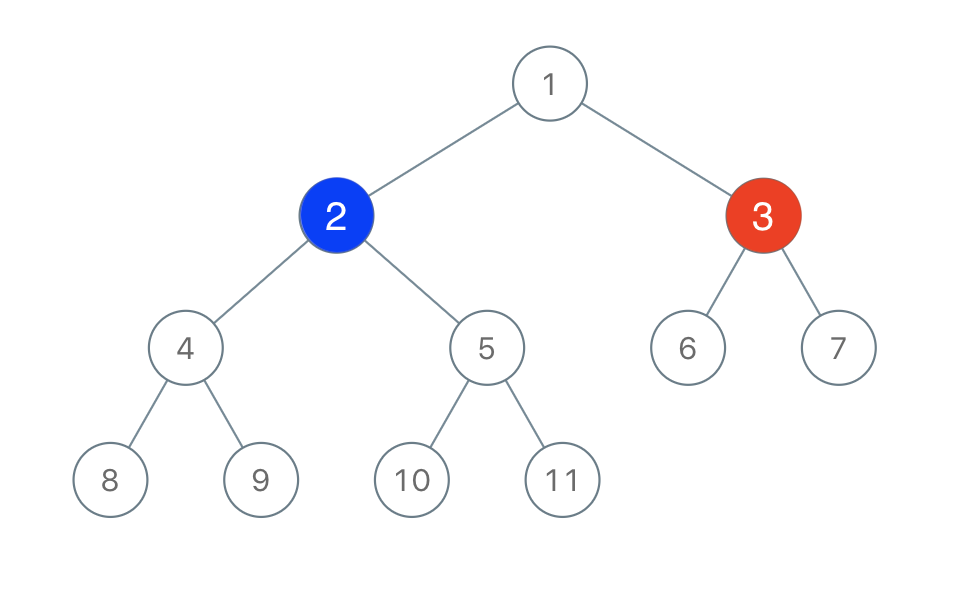# LeetCode: 1145. 二叉树着色游戏¶

## 1、题目描述¶

「一号」玩家从 [1, n] 中取一个值 x（1 <= x <= n）

「二号」玩家也从 [1, n] 中取一个值 y（1 <= y <= n）y != x

「一号」玩家给值为 x 的节点染上红色，而「二号」玩家给值为 y 的节点染上蓝色。输入：root = [1,2,3,4,5,6,7,8,9,10,11], n = 11, x = 3



• 二叉树的根节点为 root，树上由 n 个节点，节点上的值从 1 到 n 各不相同。 n 为奇数。
• 1 <= x <= n <= 100

## 2、解题思路¶

• 如何判断蓝色节点存在必胜呢？
• 初始的红色状态的节点，如果将这个节点拿掉，整个被分成了3颗子树
• 如果这3个子树的节点数量有超过一半的，那么另一个人选择了这个子树的相连节点就能获得胜利
# Definition for a binary tree node.
# class TreeNode:
#     def __init__(self, x):
#         self.val = x
#         self.left = None
#         self.right = None

class Solution:
def btreeGameWinningMove(self, root: TreeNode, n: int, x: int) -> bool:
left_red, right_red = 0, 0

def dfs(node):
nonlocal left_red, right_red
if not node:
return 0
left = dfs(node.left)
right = dfs(node.right)
if node.val == x:
left_red = left
right_red = right

return left + right + 1

dfs(root)
half = n // 2
return any([x > half for x in [(n - left_red - right_red - 1), left_red, right_red]])